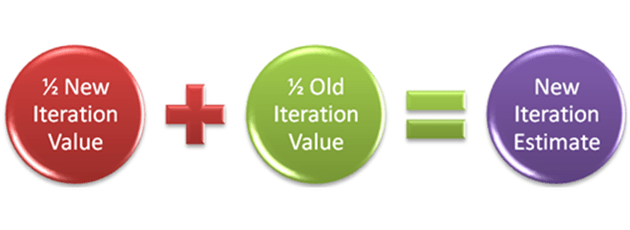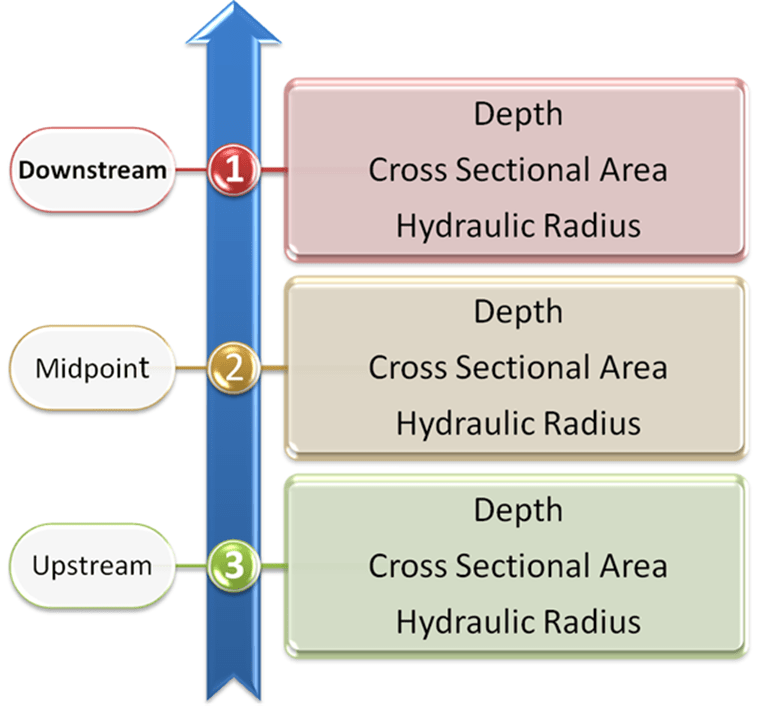H2OMAP SWMM

# Lambda Calculus and Link Variables in the #InfoSWMM, #H2OMAP SWMM and #SWMM 5 Dynamic Wave Solution

## Lambda Calculus and Link Variables in the #InfoSWMM, #H2OMAP SWMM and #SWMM 5 Dynamic Wave Solution

SWMM 5 uses the method of Successive under-relaxation to solve the Node Continuity Equation and the Link Momentum/Continuity Equation for a time step. The dynamic wave solution in dynwave.c will use up to 8 iterations to reach convergence before moving onto the next time step. The differences between the link flows and node depths are typically small (in a non pumping system) and normally converge within a few iterations unless you are using too large a time step. The number of iterations is a minimum of two with the 1st iteration NOT using the under-relaxation parameter omega. The solution method can be term successive approximation, fixed iteration or Picard Iteration, fixedpoint combinatory, iterated function and Lambda Calculus. In computer science, iterated functions occur as a special case of recursive functions, which in turn anchor the study of such broad topics as lambda calculus, or narrower ones, such as the denotational semantics of computer programs (http://en.wikipedia.org/wiki/Iterated_function).

In the SWMM 5 application of this various named iteration process there are three main concepts for starting, iterating and stopping the iteration process during one time step:

The 1st guess of the new node depth or link flow is the current link flow (Figure 3) and the new estimated node depths and link flows are used at each iteration to estimate the new time step depth or flow. For example, in the node depth (H) equation dH/dt = dQ/A the value of dQ or the change in flow and the value of A or Area is updated at each iteration based on the last iteration’s value of all node depths and link flows.

A bound or a bracket on each node depth or link flow iteration value is used by averaging the last iteration value with the new iteration value. This places a boundary on how fast a node depth or link flow can change per iteration – it is always ½ of the change during the iteration (Figure 1).

The Stopping Tolerance (Figure 2) determines how many iterations it takes to reach convergence and move out of the iteration process for this time step to the next time step.Figure 1. Under relaxation with an omega value of ½ is done on iterations 2 through a possible 20 in SWMM 5. This is not done for iteration 1.Figure 2. if the change in the Node Depth is less than the stopping tolerance in SWMM 5 the node is considered converged. The stopping tolerance is defined by the user in SWMM 5.1+.Figure 3. The differences between the link flows and node depths are typically small (in a non pumping system) and normally converge within a few iterations unless you are using too large a time step. The number of iterations is a minimum of two with the 1st iteration NOT using the under-relaxation parameter omega.

St. Venant equation – this is the link attribute data used when the St. Venant Equation is used inSWMM 5, H2OMAP SWMM and InfoSWMM. Simulated Parameters from the upstream, midpoint and downstream sections of the link are used.Figure 4. Variables Used in the St Venant Equation if used in SWMM 5.

Normal Flow Equation – this is the link attribute data used when the Normal Flow Equation is used in H2OMAP SWMM. Only simulated parameters from the upstream end of the link are used if the normal flow equation is used for the time step. The normal flow equation is used if the flow is supercritical or the water surface slope is less than the bed slope of the link.Figure 5. Variables Used in the Normal Flow Equation if used in SWMM 5.